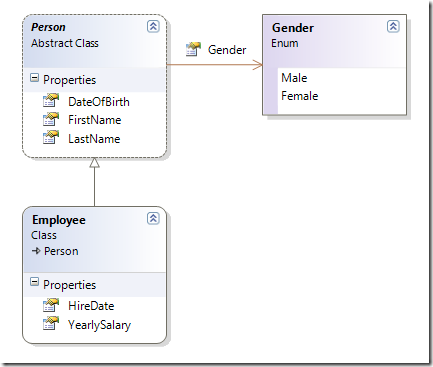# Fluent Silverlight – Fluent API and inheritance

</p>

Please view the table of content of this series for reference.

## Introduction

In my last post I showed how we  can implement a fluent API to help us construct instances of specific classes. When using a fluent API the code is in general much more readable. In this post I want to go one step further and show how we can implement a hierarchical fluent API.

## Defining a base expression

Our objects that we use in our applications are often inherited from some base class as in the following simple example.Here we have an abstract Person base class from which the Employee class inherits. We might have another class e.g. Customer that also inherits from Person. If we now want to implement a fluent API for the construction of Employee- and/or Customer objects then it makes sense that our fluent API is also hierarchically organized. We might want to build a parallel hierarchy of PersonExpression, EmployeeExpression and CustomerExpression where the latter two inherit from the first expression.

But now we have a problem. From my previous article we know that each method in a fluent API has to return the expression itself. But if we now implement say the LastName() method in the PersonExpression like this

`public abstract class PersonExpression`
`{`
`    private string lastName;`
`    ...`
` `
`    public PersonExpression LastName(string value)`
`    {`
`        lastName = value;`
`        return thisInstance();`
`    }`
` `
`    ...`
`}`
</p>

then when used in the context of an EmployeeExpression (where EmployeeExpression inherits from PersonExpression)

`Employee john = new EmployeeExpression()`
`    .FirstName("John")`
`    .LastName("Doe")`
`    ...`
</p>

we will not be able to get to any of the members defined in the EmployeeExpression any more since our continuation is of type PersonExpression and NOT of type EmployeeExpression. With other words: whenever we use a method of the base expression in our fluent expression then we loose the context!

Thus we have to find another solution. The solution is to introduce a generic parameter which will be used as the type of the return value of the methods of our expression classes. Consequently we will write</p>

`public abstract class PersonExpression<THIS>`
`    where THIS : PersonExpression<THIS>`
`{`
`    private string lastName;`
`    ...`
` `
`    public THIS LastName(string value)`
`    {`
`        lastName = value;`
`        return thisInstance();`
`    }`
` `
`    ...`
`}`
</p>

If we use the generic parameter THIS instead of a concrete (base) expression, then we will never loose the context. Please note also that the LastName method does not return ‘this’ any more but rather the return value of the function thisInstance which is defined as follows

`protected THIS thisInstance()`
`{`
`    return (THIS)this;`
`}`
</p>

So we never should return directly this any more in our expression hierarchy but rather thisInstance().

But wait, we have still one more problem: since we want to define the implicit type conversion in the base class we also have to introduce the target type as a generic parameter such as that we can then write

`public static implicit operator TARGET(PersonExpression<THIS, TARGET> expression)`
`{`
`    var target = new TARGET();`
`    expression.BuildUp(target);`
`    return target;`
`}`
</p>

where the BuildUp function is defined as follows

`protected virtual void BuildUp(TARGET target)`
`{`
`    ...`
`}`
</p>

this method will be overriden by the child expressions to build-up the target object.</p>

The full code for the PersonExpression will now look like this

`public abstract class PersonExpression<THIS, TARGET>`
`    where THIS : PersonExpression<THIS, TARGET>`
`    where TARGET : Person, new()`
`{`
`    private string lastName;`
`    private string firstName;`
`    private DateTime dateOfBirth;`
`    private Gender gender;`
` `
`    public THIS LastName(string value)`
`    {`
`        lastName = value;`
`        return thisInstance();`
`    }`
` `
`    public THIS FirstName(string value)`
`    {`
`        firstName = value;`
`        return thisInstance();`
`    }`
` `
`    public THIS DateOfBirth(DateTime value)`
`    {`
`        dateOfBirth = value;`
`        return thisInstance();`
`    }`
` `
`    public GenderExpression<PersonExpression<THIS, TARGET>> Gender`
`    {`
`        get{ return new GenderExpression<PersonExpression<THIS, TARGET>>(this, x => gender = x);}`
`    }`
` `
`    protected THIS thisInstance()`
`    {`
`        return (THIS)this;`
`    }`
` `
`    protected virtual void BuildUp(TARGET target)`
`    {`
`        target.LastName = lastName;`
`        target.FirstName = firstName;`
`        target.DateOfBirth = dateOfBirth;`
`        target.Gender = gender;`
`    }`
` `
`    public static implicit operator TARGET(PersonExpression<THIS, TARGET> expression)`
`    {`
`        var target = new TARGET();`
`        expression.BuildUp(target);`
`        return target;`
`    }`
`}`
</p>

Note how the two generic parameters are restricted by their respective where filters. This restriction is very important to make the whole concept work.

The full code for the EmployeeExpression is given below

`public class EmployeeExpression : PersonExpression<EmployeeExpression, Employee>`
`{`
`    private DateTime hireDate;`
`    private decimal yearlySalary;`
` `
`    public EmployeeExpression HireDate(DateTime value)`
`    {`
`        hireDate = value;`
`        return thisInstance();`
`    }`
` `
`    public EmployeeExpression YearlySalary(decimal value)`
`    {`
`        yearlySalary = value;`
`        return thisInstance();`
`    }`
` `
`    protected override void BuildUp(Employee target)`
`    {`
`        base.BuildUp(target);`
`        target.HireDate = hireDate;`
`        target.YearlySalary = yearlySalary;`
`    }`
`}`
</p>

and the expression can now be used in the following way

`Employee john = new EmployeeExpression()`
`    .FirstName("John")`
`    .LastName("Doe")`
`    .DateOfBirth(new DateTime(1964, 4, 14))`
`    .HireDate(new DateTime(2007, 3, 1))`
`    .YearlySalary(90000);`
</p>

With the aid of some “generics magic” we are now able to implement a hierarchically structured fluent API for our objects we want to construct. This is a very important pattern we used when implementing Fluent Silverlight.